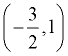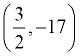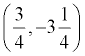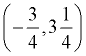Quadratic Graph Practice Terms of Use    Contact Person: Donna RobertsDirections: Grab your paper and pencil. Write a solution to the following problems. Be sure to show your work to support your answer. Use your graphing calculator for checking only.

 1. Consider the equation y = x2 - x - 6. Answer the following questions, stating how you arrived at your answer. a) Determine whether the parabola opens upward or downward. b) Find the y-intercept. c) Find the x-intercepts, if any. d) Find the equation of the axis of symmetry. e) Sketch the graph.

2.
Given a quadratic function, which of the following choices explains the meaning of the zeros graphically.
Choose:
 When factored, f (x) = (x - p)(x - q) where p and q correspond to the y-intercepts. When factored, f (x) = (x - p)(x - q) where p and q correspond to the x-intercepts. When factored, f (x) = (x - p)(x - q) where p and q correspond to the turning points of the graph. When factored, f (x) = (x - p)(x - q) where p and q correspond to the axis of symmetry.

 3. Which of the following equations could describe the function seen in the graph at the right? (Check all that apply!) y = (x + 2)(x - 5) y = -2x2 + 4x - 1 y = (x - 6)(x - 10) y = (x + 5)2 + 4 y = (x - 8)2 - 6 y = (15 - x)(10 - x) y = (-x + 3)(x - 5)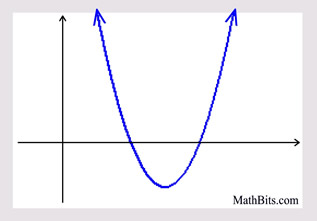4.
Shown below is the equation for function f (x), and the graph of parabolic function g (x). Which function has the larger maximum?
 Choose: f (x) g (x)
 f (x) = -(x - 4)2 + 5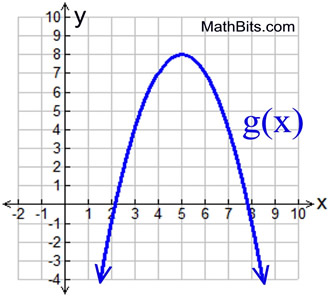5.
What is the equation of the axis of symmetry for this parabola?
Choose:
 x = 2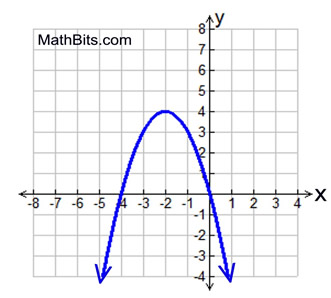y = 4 x = -2 y = -2

6.
Which of the following statements is NOT true for the parabola seen in the graph?
Choose:
 The axis of symmetry is x = 1.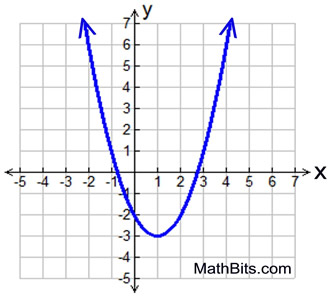The vertex is (1,-3). The "a" coefficient is positive. The parabola has two positive roots.

7.
What is the equation of the axis of symmetry of the graph:
y = 3x2 + 6x - 1
Choose:
 x = 2 x = -1 x = 1 x = -2

8.
Which equation could be the equation of the graphed parabola?
Choose:
 y = -x2 - 4x - 5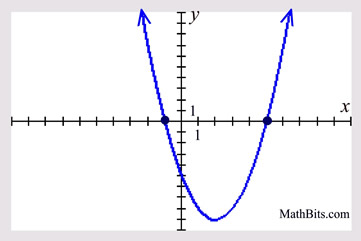y = -x2 + 4x - 5 y = x2 - 4x - 5 y = x2 + 4x - 5

9.
What are the roots of the graphed parabola, assuming the roots to be integers?
Choose:
 x = ± 2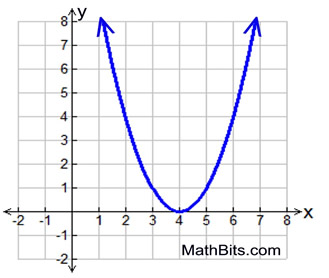x = ± 4 x = 2; x = 2 x = 4; x = 4

10.
What is the vertex, or turning point, of the parabola y = 4x2 - 6x - 1?
Choose: